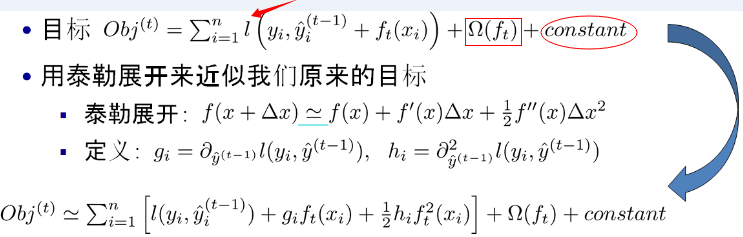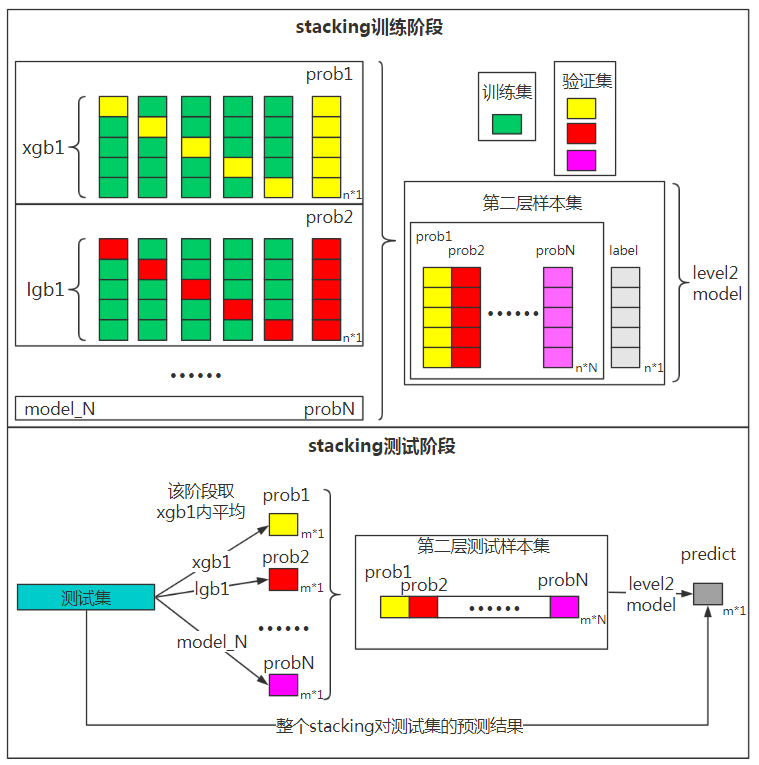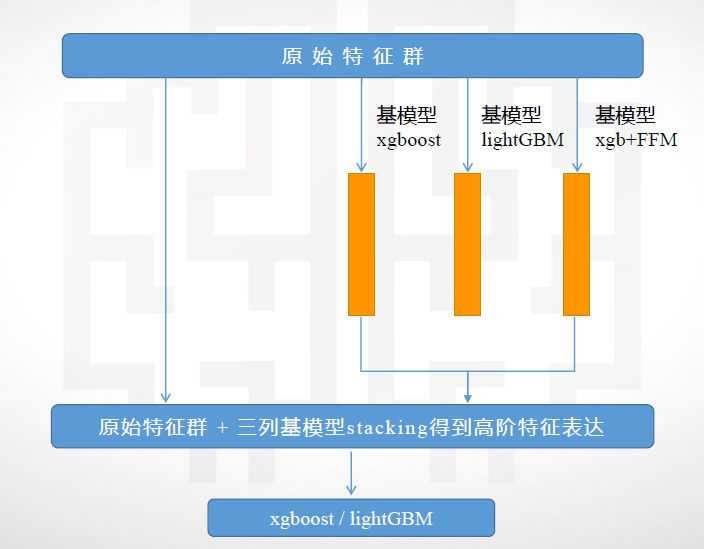# python机器学习案例系列教程——集成学习（Bagging、Boosting、随机森林RF、AdaBoost、GBDT、xgboost）

python数据挖掘系列教程

## 偏差与方差

“偏差-方差分解”（bias variance decomposition）是用来解释机器学习算法的泛化能力的一种重要工具。对于同一个算法，在不同训练集上学得结果可能不同。对于训练集$D=\left({x}_{1},{y}_{1}\right),\left({x}_{2},{y}_{2}\right),\cdots ,\left({x}_{N},{y}_{N}\right)$$D={(x_1 ,y_1 ),(x_2 ,y_2 ),⋯,(x_N ,y_N )}$，由于噪音，样本$x$$x$的真实类别为$y$$y$（在训练集中的类别为$y$$y$），则噪声为

${\xi }^{2}={E}_{D}\left[\left({y}_{d}-y{\right)}^{2}\right]$

$\stackrel{^}{f\left(x\right)}={E}_{D}\left[f\left(x;D\right)\right]$

$var\left(x\right)={E}_{D}\left[\left(f\left(x;D\right)-\stackrel{^}{f\left(x\right)}{\right)}^{2}\right]$

$bia{s}^{2}\left(x\right)=\left(\stackrel{^}{f\left(x\right)}-y{\right)}^{2}$

## Bagging

Bagging（Bootstrap Aggregating）对训练数据采用自助采样（boostrap sampling），即有放回地采样数据；每一次的采样数据集训练出一个基分类器，经过M次采样得到M个基分类器，然后根据最大表决（majority vote）原则组合基分类器的分类结果。## Boosting

Boosting的思路则是采用重赋权（re-weighting）法迭代地训练基分类器，即对每一轮的训练数据样本赋予一个权重，并且每一轮样本的权值分布依赖上一轮的分类结果；基分类器之间采用序列式的线性加权方式进行组合。他通过迭代地训练一系列的分类器，每个分类器采用的样本分布都和上一轮的学习结果有关。其代表算法是AdaBoost， GBDT。## Bagging、Boosting二者之间的区别

Bagging：训练集是在原始集中有放回选取的，从原始集中选出的各轮训练集之间是独立的。
Boosting：每一轮的训练集不变，只是训练集中每个样例在分类器中的权重发生变化。而权值是根据上一轮的分类结果进行调整。

Bagging：使用均匀取样，每个样例的权重相等。
Boosting：根据错误率不断调整样例的权值，错误率越大则权重越大。

Bagging：所有预测函数的权重相等。
Boosting：每个弱分类器都有相应的权重，对于分类误差小的分类器会有更大的权重。

Bagging：各个预测函数可以并行生成。
Boosting：各个预测函数只能顺序生成，因为后一个模型参数需要前一轮模型的结果。

## 总结

Bagging + 决策树 = 随机森林
AdaBoost + 决策树 = 提升树
Gradient Boosting + 决策树 = GBDT

## 随机森林RF

1）对于$t=1,2...,T$$t=1,2...,T$

• a)对训练集进行第t次随机采样，得到一个包含m个样本的采样集${D}_{m}$$D_m$
• b)用采样集${D}_{m}$$D_m$训练第m个决策树模型${G}_{m}\left(x\right)$$G_m(x)$，在训练决策树模型的节点的时候， 在所有的特征中选择一部分特征，在这些随机选择的部分样本特征中选择最优的特征来做决策树的左右子树划分。

2) 如果是分类算法预测，则T个弱学习器投出最多票数的类别或者类别之一为最终类别。如果是回归算法，T个弱学习器得到的回归结果进行算术平均得到的值为最终的模型输出。

RF的主要优点有：

1）训练可以高度并行化，对于大数据时代的大样本训练速度有优势。个人觉得这是的最主要的优点。
2）由于可以随机选择决策树节点划分特征，这样在样本特征维度很高的时候，仍然能高效的训练模型。
3）在训练后，可以给出各个特征对于输出的重要性
4）由于采用了随机采样，训练出的模型的方差小，泛化能力强。
5）相对于Boosting系列的Adaboost和GBDT， RF实现比较简单。
6）对部分特征缺失不敏感。

RF的主要缺点有：

1)在某些噪音比较大的样本集上，RF模型容易陷入过拟合。
2)取值划分比较多的特征容易对RF的决策产生更大的影响，从而影响拟合的模型的效果。

## AdaBoost

AdaBoost是由Freund与Schapire提出来解决二分类问题$y\in \left\{1,-1\right\}$$y∈\{1,-1\}$，其定义损失函数为指数损失函数：

$L\left(y,f\left(x\right)\right)=exp\left(-yf\left(x\right)\right)$

$L\left(y,f\left(x\right)\right)=\frac{1}{n}\sum _{i=1}^{n}exp\left(-{y}_{i}f\left({x}_{i}\right)\right)$

${f}_{m}\left(x\right)={f}_{m-1}\left(x\right)+{\alpha }_{m}{G}_{m}\left(x\right)$

${\alpha }_{m}=\frac{1}{2}log\frac{1-{e}_{m}}{{e}_{m}}$

${e}_{m}=\frac{\sum _{i=1}^{N}{w}_{i}^{\left(m\right)}I\left({y}_{i}\ne G\left({x}_{i}\right)\right)}{\sum _{i=1}^{N}{w}_{i}^{\left(m\right)}}$

$m+1$$m+1$轮的训练数据集权值分布
${D}_{m+1}=\left({w}_{m+1},1,\cdots ,{w}_{m+1},i,\cdots ,{w}_{m+1},N\right)$$D _{m+1} =(w_{m+1},1 ,⋯,w_{m+1},i ,⋯,w_{m+1},N )$

${w}_{m+1,i}=\frac{{w}_{m,i}}{{Z}_{m}}exp\left(-{\alpha }_{m}{y}_{i}{G}_{m}\left({x}_{i}\right)\right)$

${Z}_{m}=\sum _{i=1}^{N}{w}_{m,i}\ast exp\left(-{\alpha }_{m}{y}_{i}{G}_{m}\left({x}_{i}\right)\right)$

$sign\left(f\left(x\right)\right)=sign\left(\sum _{m=1}^{M}{\alpha }_{m}{G}_{m}\left(x\right)\right)$

${\alpha }_{m}$$α_m$${e}_{m}$$e_m$的单调递减函数，特别地，当${e}_{m}\le 0.5$$e_m ≤0.5$时，${\alpha }_{m}$$α_m$ ≥0；当${e}_{m}>12$$e_m >12$时，即基分类器不满足弱可学习的条件（比随机猜测好），则应该停止迭代。

${D}_{1}\left(i\right)=1/N$$D_1 (i)=1/N$ %初始化权重分布
for m=1,⋯,M m=1,⋯,M:
learn base classifier ${G}_{m}\left(x\right)$$G_m(x)$;
if ${e}_{m}>0.5$$e_m>0.5$ then break；
update ${\alpha }_{m}$$α_m$and ${D}_{m+1}$$D_{m+1}$;
end for

AdaBoost能够自适应（addaptive）地调整样本的权值分布，将分错的样本的权重设高、分对的样本的权重设低；所以被称为“Adaptive Boosting”。

sklearn的AdaBoostClassifier实现了AdaBoost，默认的基分类器是能fit()带权值样本的DecisionTreeClassifier。

$L\left(y,f\left(x\right)\right)=\frac{1}{n}\sum _{i=1}^{n}exp\left(-{y}_{i}f\left({x}_{i}\right)\right)$

$\sum _{i=1}^{n}{\overline{w}}_{mi}{e}^{{y}_{i}{\alpha }_{t}{G}_{t}\left({x}_{i}\right)}\phantom{\rule{0ex}{0ex}}=\sum _{{y}_{i}={G}_{t}\left({x}_{i}\right)}{\overline{w}}_{mi}{e}^{-{\alpha }_{m}}+\sum _{{y}_{i}\ne {G}_{m}\left({x}_{i}\right)}{\overline{w}}_{mi}{e}^{{\alpha }_{m}}\phantom{\rule{0ex}{0ex}}=\left({e}^{{\alpha }_{m}}-{e}^{-{\alpha }_{m}}\right)\sum _{i=1}^{n}{\overline{w}}_{mi}I\left({y}_{i}\ne {G}_{m}\left({x}_{i}\right)\right)+{e}^{-{\alpha }_{m}}\sum _{i=1}^{n}{\overline{w}}_{mi}$

${\alpha }_{m}^{\ast }=\frac{1}{2}log\frac{1-{e}_{m}}{{e}_{m}}$

${e}_{m}=\frac{\sum _{i=1}^{n}{\overline{w}}_{mi}I\left({y}_{i}\ne {G}_{m}\left({x}_{i}\right)\right)}{\sum _{i=1}^{n}{\overline{w}}_{mi}}\phantom{\rule{0ex}{0ex}}=\sum _{i=1}^{n}{w}_{mi}I\left({y}_{i}\ne {G}_{m}\left({x}_{i}\right)\right)$

https://github.com/626626cdllp/data-mining/tree/master/ensemble-learning/adaboost

## GBDT梯度提升决策树

gbdt全称梯度下降树，在传统机器学习算法里面是对真实分布拟合的最好的几种算法之一。

GBDT(Gradient Boosting Decision Tree) 又叫 MART（Multiple Additive Regression Tree)，是一种迭代的决策树算法，该算法由多棵决策树组成，所有树的结论累加起来做最终答案。它在被提出之初就和SVM一起被认为是泛化能力较强的算法。

GBDT中的树是回归树（不是分类树），GBDT用来做回归预测，调整后也可以用于分类。

1、初始化，估计使损失函数极小化的常数值，它是只有一个根节点的树，即ganma是一个常数值。
2、
（a）计算损失函数的负梯度在当前模型的值，将它作为残差的估计
（b）估计回归树叶节点区域，以拟合残差的近似值
（c）利用线性搜索估计叶节点区域的值，使损失函数极小化
（d）更新回归树
3、得到输出的最终模型 f(x)GBDT通过多轮迭代,每轮迭代产生一个弱分类器，每个分类器在上一轮分类器的残差基础上进行训练。对弱分类器的要求一般是足够简单，并且是低方差和高偏差的。因为训练的过程是通过降低偏差来不断提高最终分类器的精度。

## xgboost## 模型融合：bagging、Boosting、Blending、Stacking

1、Bagging：使用训练数据的不同随机子集来训练每个 Base Model，最后进行每个 Base Model 权重相同的 Vote。也即 Random Forest 的原理。
2、Boosting：迭代地训练 Base Model，每次根据上一个迭代中预测错误的情况修改训练样本的权重。也即 Gradient Boosting，Adaboost 的原理。比 Bagging 效果好，但更容易 Overfit。
3、Blending：用不相交的数据训练不同的 Base Model，将它们的输出取（加权）平均。实现简单，但对训练数据利用少了。
4、Stacking：